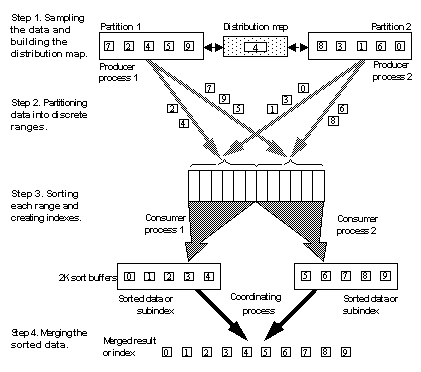# Overview of the parallel sorting strategy

Like the Adaptive Server optimizer, the Adaptive Server parallel sort manager analyzes the available worker processes, the input table, and other resources to determine the number of worker processes to use for the sort.

After determining the number of worker processes to use, Adaptive Server executes the parallel sort. The process of executing a parallel sort is the same for create index commands and queries that require sorts. Adaptive Server executes a parallel sort by:

1. Creating a distribution map. For a merge join with statistics on a join column, histogram statistics are used for the distribution map. In other cases, the input table is sampled to build the map.

2. Reading the table data and dynamically partitioning the key values into a set of sort buffers, as determined by the distribution map.

3. Sorting each individual range of key values and creating subindexes.

4. Merging the sorted subindexes into the final result set.

Each of these steps is described in the sections that follow.

Figure 26-1 depicts a parallel sort of a table with two partitions and two physical devices on its segment.

Figure 26-1: Parallel sort strategy